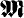# Measure of a Set

The following article is from The Great Soviet Encyclopedia (1979). It might be outdated or ideologically biased.

## Measure of a Set

a mathematical concept that generalizes the concept of the length of a segment, the area of a plane figure, and the volume of a solid to sets of a more general nature. As an example we cite the definition of measure for bounded plane sets introduced by H. Lebesgue in 1902. In defining the Lebesgue measure of a set, just as in defining the area of plane figures in geometry, we compare the part of the plane occupied by the set with the selected unit of measurement. The comparison process is similar to the ordinary process of measuring area. The Lebesgue measure m(Δ) of any square Δ is assumed to be equal to the area of the square. The set A under consideration is covered with a finite or countably infinite number of squares Δ1, Δ2, … , Δn, … . The least upper bound of the numbers Σn=1 mn) taken over all possible covers of the set A is called the outer measure m*(A) of set A. The inner measure m*(A) of A is defined as the difference m(Δ) − m*(Ā), where Δ is some square that contains A and Ā is the set of all points of this square that do not belong to A.

Sets for which the outer measure is equal to the inner measure are said to be Lebesgue measurable, and the common value m(A) of the outer and inner measures is called the Lebesgue measure of A. Geometric figures that have an area in the elementary sense are measurable, and their Lebesgue measure coincides with their area. However, there exist measurable sets that have no area.

Lebesgue measure on the line can be defined in a similar manner. Here, the outer measure is determined by considering all finite and countable coverings of a set by means of intervals.

The main properties of Lebesgue measure are (1) the measure of any set is non-negative— m(A) ≥ 0; (2) the measure of the union A = Σn=1 An of a finite or countable system of pairwise disjoint sets A1, A2, … , An,…. is equal to the sum of the measures of the component sets— m(A) = Σn=1 m (An; and (3) the measure of a set does not change under a rigid motion.

The distinctiveness of the concept of measure of a set may be clarified by the following example. The set A of rational points on the interval (0,1) and the set B of irrational points on the same interval are similar in that each of them is dense in that interval, that is, both points of A and points of B can be found between any two points of this interval; at the same time, A and B greatly differ in measure: m(A) = 0 and m(B) = 1.

A measure coincident with the Lebesgue measure was defined before Lebesgue by M. E. C. Jordan (1893) and E. Borel (1898) for narrower classes of sets.

The development of a number of branches of modern mathematics has led to further generalizations—the creation of what is called abstract measure theory. Here, the measure of a set is defined axiomatically. Let U be an arbitrary set and letbe some family of its subsets. The non-negative function μ(A), defined for all A belonging tois called a measure if it is completely additive [that is, if for any sequence of disjoint sets A1,A2,…, An, … belonging to, whose union A belongs to, the equality μ(A)-Σn=1 μ(An holds] and if, moreover, the systemsatisfies certain additional conditions. Sets belonging toare said to be measurable (with respect to the measure JUL). After the measure γ is defined, the concept of functions measurable with respect to γ and the operation of integration are introduced.

Many fundamental assertions of the theory of Lebesgue measure, the theory of measurable functions, and the theory of the Lebesgue integral are preserved with appropriate changes, in the abstract theory of measure and integral. This theory constitutes the mathematical foundation of modern probability theory, which was given by A. N. Kolmogorov in 1933. Measures tha are invariant with respect to a given group of mappings of the set U into itself are of particular interest for a number of fields of mathematics.

### REFERENCES

Kolmogorov, A. N., and Fomin, S. V. Elementy teorii funktsii i funktsional’rnogo analiza, 3rd ed. Moscow, 1972.
Lebesgue, H. Integrirovanie i otyskanie primitivnykh funktsii. Moscow-Leningrad, 1934. (Translated from French.)
Saks, S. Teoriia integrala. Moscow, 1949. (Translated from English.)
Halmos, P. R. Teoriia mery. Moscow, 1953. (Translated from English.)

IU. V. PROKHOROV

The Great Soviet Encyclopedia, 3rd Edition (1970-1979). © 2010 The Gale Group, Inc. All rights reserved.
Site: Follow: Share:
Open / Close